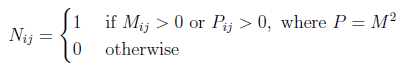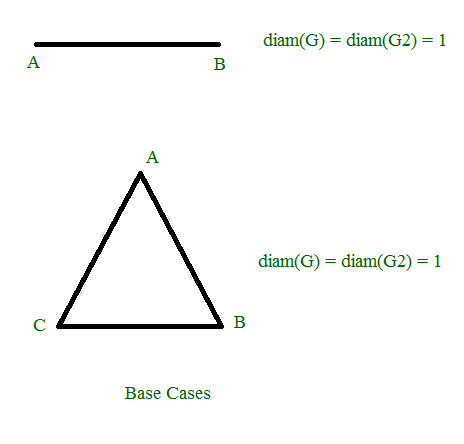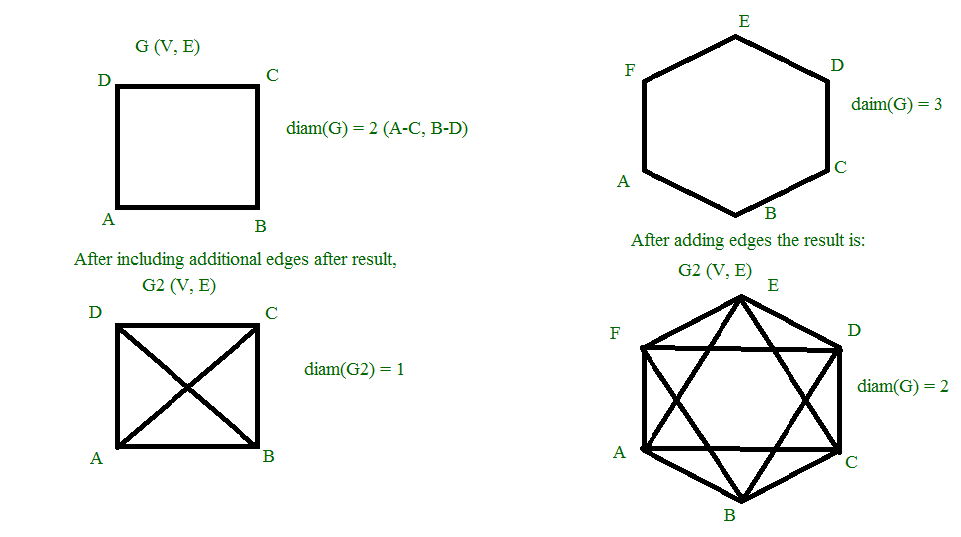# GATE | GATE CS 2021 | Set 1 | Question 46

• Difficulty Level : Expert
• Last Updated : 08 Jul, 2021

Let G=(V,E) be an undirected unweighted connected graph. The diameter of G is defined as:Let M be the adjacency matrix of G.

Define graph G2 on the same set of vertices with adjacency matrix N, whereWhich one of the following statements is true?
(A) diam(G2)≤⌈ diam(G)/2⌉
(B) ⌈ diam(G)/2⌉2)< diam(G)
(C) diam(G2) = diam(G)
(D) diam(G)< diam(G2)≤2 diam(G)

Explanation: M2 will be the adjacency matrix of the graph H derived from G as follow:

```H(a,b) = 1 iff (there exists a vertex c st. G(a,c) = 1 and G(c,b) = 1)
```

So basically there is an edge between a,b iff there is some vertex c st. there is an edge (a,c) and an edge (c,b) in G.
When we do G∪H in the resulting graph diameter reduces to 0.5 of the original graph.

Alternative :

The given graph G is always be a connected graph (as mentioned).

As the graph is unweighted graph the shortest path between vertices is the minimum number of edges to travel through. Given M is the adjacency matrix of graph G and N is the adjacency matrix of graph G2, The cells in matrix N hold the condition that it has value 1 if Mij > 0 or M2ij > 0 and 0 otherwise.

As a base case for a graph G for two or three vertices diam(G) will be 1 since the shortest path is 1 unit far from each vertex. Thereafter graph G2 to be formed from those vertices situated at their respective positions. The Graph G2 is the same as G. Hence diam(G) = diam(G2)  {  for | V | = {2,3}  }for | V | in { 3, 6 } As shown, The square of the matrix M ( P ) in union with M, ensures the additional edges between the vertices which are 2 units far from each other.In the first figure for | V | = 4, diam(G) = 2 ( vertices A and C are 2 units far from each other ) after squaring the adjacency matrix M we get additional cells getting 1 in matrix N which includes edges between those two vertices which are two units far from each other i.e edge AC and BD. Thus diam(G2) = 1. Since all vertices can be visited with at most 1 unit.

Similarly for the graph of | V | = 6, diam(G) = 3 ( vertices B and E are 3 units far from each other ) after squaring the 6 X 6 matrix we get P( M2 ) which involves additional edges BD, DF, FB, CE, EA, AC. Thus all the vertices can be visited with at most 2 units. Hence diam(G2) = 2

Thus here if P would have been given nth power of M, then the edges between those two vertices which are n units far from each other will be added. ( if not present in graph G already ) Thus in general, diam(G2) <= ceil( diam(G) / n )

My Personal Notes arrow_drop_up
Recommended Articles
Page :